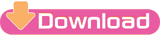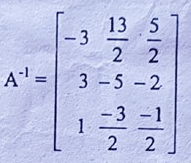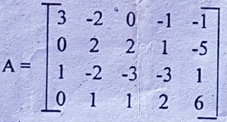2076 (2019)
BACHELOR LEVEL (4 Yrs.)/EDUCATION/IV Year
Linear Algebra and Vector Analysis (Math.Ed.445) Major
Time: 3 hrs
Full Marks: 100
DOWNLOAD PDF | Bachelor Level 4 Years Education (IV Year) - Linear Algebra and Vector Analysis | Math.Ed.445 | Question Paper | 2076/2019Also Check:
Check and Download | All Question Papers Of Bachelor 4 Years Education (IV Years) | 2076/2019
Candidates are required to give their answers in their own words as far as practicable.
The figures in the margin indicate full marks.
Attempt ALL the questions.
Group 'B'    [8x7=56]
1. Find the matrix A whose inverse isFind the matrix A?
OR
Solve the following equations by using Cramer's rule.
x+2y+3z=6
2x+4y+z=7
3x+2y+9z=14

2. Find the rank of the matrixFind rank of the matrix?
by reducing the matrix to Echelon form.

3. Determine the scalar and vector projection of B onto A if A=(1,2,3) and B=(-1,2,-3),

OR
Prove that the union of two subspaces of a vector space is a subspace if and only if one is contained in the other.

4. Define convex sub set of a vector space. Let P1, P2, ....., Pn be points of a vector space V. Let S be the set of all linear combinations t1+t2+.....+tn=1. Then prove that S is convex.

5. Solve the equation x4-2x2+8x-3=0 by Descartes' method.

View the question paper of Linear Algebra and Vector Analysis (Math.Ed.445) | Bachelor Level (4 Yrs.) | Education | IV Years | TULinear Algebra and Vector Analysis (Math.Ed.445) Major 2076/2019 Question Paper Bachelor Level (4 Yrs.) Education IV Year TULinear Algebra and Vector Analysis (Math.Ed.445) Major 2076/2019 Question Paper Bachelor Level (4 Yrs.) Education IV Year TU